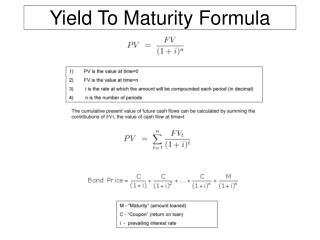Download PresentationYield To Maturity Formula

Loading in 2 Seconds...

# Yield To Maturity Formula - PowerPoint PPT Presentation

Yield To Maturity Formula. Yield to Maturity Equation - Closed Form (started with a geometric series…). Calculate price of bond with par value of \$1,000 to be paid in 10 years, a coupon of 10% and YTM of 12%. Assume coupons are paid semi-annually to bond holders:I am the owner, or an agent authorized to act on behalf of the owner, of the copyrighted work described.
Download Presentation## Yield To Maturity Formula

An Image/Link below is provided (as is) to download presentation

Download Policy: Content on the Website is provided to you AS IS for your information and personal use and may not be sold / licensed / shared on other websites without getting consent from its author.While downloading, if for some reason you are not able to download a presentation, the publisher may have deleted the file from their server.

- - - - - - - - - - - - - - - - - - - - - - - - - - E N D - - - - - - - - - - - - - - - - - - - - - - - - - -
Presentation Transcript
1. Yield To Maturity Formula

2. Yield to Maturity Equation - Closed Form (started with a geometric series…) Calculate price of bond with par value of \$1,000 to be paid in 10 years, a coupon of 10% and YTM of 12%. Assume coupons are paid semi-annually to bond holders: Determine number of coupon payments (2 per year for 10 years = 20) Determine value of each coupon payment (divide coupon in half since semi-annual). Each payment will be \$50 (\$1000 * 0.05). Determine the semi-annual yield: Like the coupon rate, the YTM of 12% must be divided by 2.

3. YTM - Frequency Parameterized Accounting for different payment frequencies: Most bonds pay semi-annually but to make our formula more general we extend formula with parameter “F” below. if a bond was paying annual coupons F = 1, quarterly = 4

4. While more programmer friendly, what do we assume away with this formula? • Assumes all intermediate flows are discounted at same rate (the YTM) • A more general implementation is the “cash-flow series” • A collection of individual cash-flows which can: • Support assigning different interest rates to individual cash-flows • Represent changing principal amounts (e.g., for amortizing instruments) • Support independent processing of individual cash-flows (e.g., date adjustments)

5. Deliverables for next week: • Download the “living spec” prototype spreadsheets. • Build calculator class around PV function • Implement Closed Form Equation • Calculate price from yield and price sensitivity to yield change (first partial derivative) : “DV01” (dollar value of 1/100 of a percent) • Download sample code to load test data • File access & parsing “SBB_util.*” • Run your main() loading data.txt on site. • You will need to calculate number of periods… (download the date code on the site)